Next: Outlook Up: Measurement Results Previous: Beam Polarization Level   Contents

## Beam Energy

In order to perform the energy calibration measurement a decent polarization build-up had to be observed as mentioned in the last chapter. Afterwards an orbit correction was done to ensure that the orbit had zero deviation from the design orbit thus the measurement would reveal the actual beam energy not including possible distortion due to corrector settings modifying the integrated bending field. Then the sweep could start within the predefined range and dwell settings. Beam current, lifetime, loss monitor reading and actual sweep frequency were monitored as shown in figure 9.

In a first measurement run we performed a frequency sweep from 2.38 GeV (417.833 kHz) to 2.4 GeV (465.112 kHz) with a dwell of 10 Hz/s and a kicker power of 135 W. The product of beam current and lifetime was flat, thus showing that the resonant frequency had not been reached in this sweep. We decided to start another sweep above 2.4 GeV.

In the second measurement the sweep went from 2.4 GeV (465.112 kHz) to 2.42 GeV (512.391 kHz) with the same power and dwell settings. The result of this run is shown in figure 13. Two effects were observed: At time stamp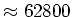s a dip in the product of beam current and lifetime is noticed due to a lifetime drop by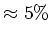. This dip is located at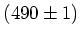kHz which corresponds to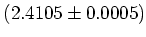GeV, but due to the mirror uncertainty (as already mentioned in chapter 3.4), could also be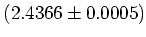GeV. In a second measurement this dip was reproduced, but due to a vacuum interlock the identification of the mirror had to be postponed. The second important effect seen in figure 13 are the three peaks; the central peak is a (not yet identified) orbital resonance with two sidebands. The sidebands are located at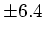kHz from the center resonance which corresponds to a synchrotron tune of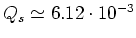. An interesting fact is that to the right of the the depolarizing dip the plotted curve isn't flat as one would expect from the resonance crossing formula of Froissart and Stora : After the resonance dip a new build-up is observed with the same exponential build-up characteristics as observed in equilibrium polarization level measurements (see also figure 19).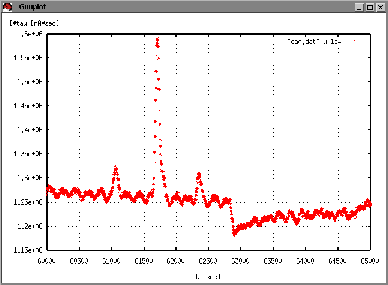Figure 13: First sweep around a depolarizing resonance. The resonance is located at 62800 s whereas the three peaks come from an orbital resonance; a central resonance and its first order sidebands. The distance between center resonance and sidebands is the synchrotron tune.

In a third run we tried to reproduce the dip above the half number (the half number spin tune corresponds to 520.833 kHz). The sweep went from 523 kHz to 591 kHz with a dwell of 18 Hz/s and 250 W kicker power. The result is shown in figure 14. A clear dip shows that the depolarizing resonance lies at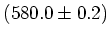kHz corresponding to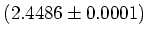GeV or the mirror at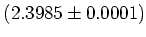GeV. This dip is confirmed in the peak of loss monitor coincidence signals as depicted in figure 15. It was however observed at the end of the sweep that the mean orbit had drifted, thus causing the energy to change. Therefore the measurement had to be repeated.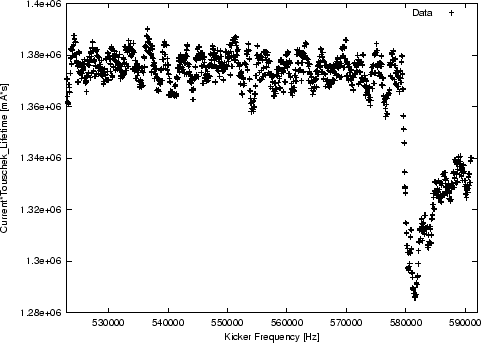Figure 14: The depolarizing resonance at 580kHz.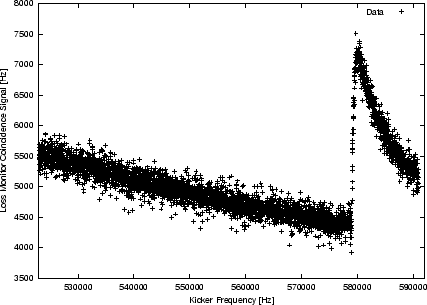Figure 15: The loss monitor coincidence signal rising during resonant depolarization at 580kHz.

In a fourth run the last measurement was verified: In a fast sweep (dwell was 30 Hz/s) from 523 kHz to 607 kHz a pattern of dips was detected starting at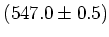kHz. This is shown in figure 16. A second dip is at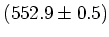kHz and a third at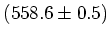kHz; afterwards the orbit resonance leads to the three peaks already seen in earlier experiments - they are again separated by the synchrotron tune. Note that the orbit resonance peaks are to the right of the depolarizing resonance compared to figure 13 where they were to the left. This has to do with the fact that we are now sweeping above the half-integer so our view is mirrored''.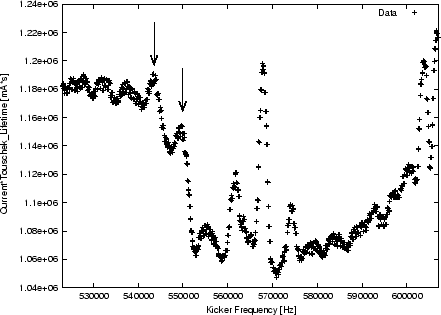Figure 16: Dip pattern of a fast sweep.

The three dips correspond to the energies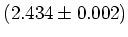GeV (mirror at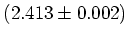GeV),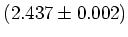GeV (mirror at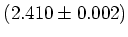GeV) and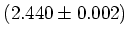GeV (mirror at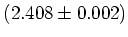GeV). The question arises which of these dips is the resonance and which are sidebands as well as is this the resonance or just its mirror? To answer these questions two identical runs were started differing only by a changed RF voltage. Prior to the second sweep the RF voltages were increased from 1752.1 kV to 1944.8 kV (+11%). According to equation 53 this should lead to a change of the synchrotron tune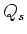by more than a percent which can be observed easily. The patterns of the two sweeps are compared in figure 17.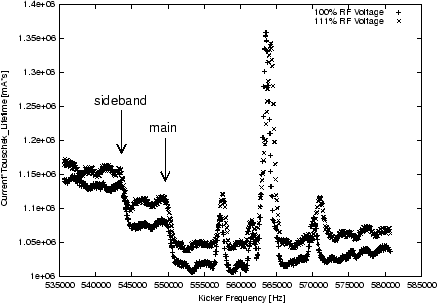Figure 17: Dip patterns of two sweeps differing by a slightly changed RF voltage.

The synchrotron tune increased byand one dip could be identified to have not moved within the precision of measurement. Another dip (to the left of the resonance) was shifted by 5% to lower frequencies while a dip above the resonance was shifted to higher frequencies, precisely as predicted by theory. The resonance is located at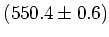kHz corresponding to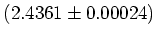GeV (mirror at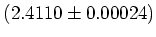GeV).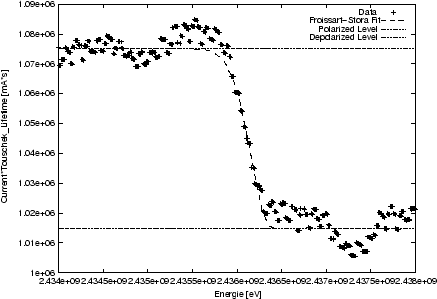Figure 18: Dip of the resonance with fit according to the Froissart-Stora equation for resonance-crossing.

A magnified view of the resonance dip is given in figure 18. Notice the fit curve from the upper level (polarized beam) to the lower level (partially depolarized beam) according to the Froissart-Stora equation . Froissart and Stora described the curve that would be observed when crossing a depolarizing resonance and claimed it to be: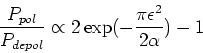(58)

where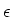is the strength of the resonance and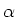the resonance crossing speed. The slow build-up after crossing the resonance is due to the fact that the kicker magnet has already depolarized a large fraction of the beam while being in the area of the resonance. When the sweep reaches values outside of the resonance a slow polarization build-up starts again thus leading to a almost flat curve. A very nice example for this build-up process after crossing the depolarizing resonance is shown in figure 19.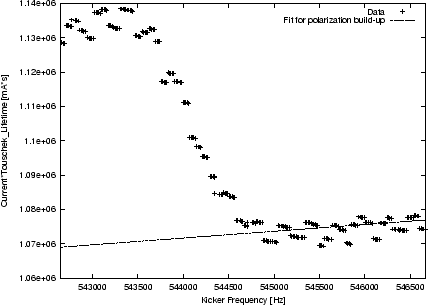Figure 19: Slow polarization build-up process after having crossed the depolarizing resonance.

The exact energy calibration is obtained with the Froissart-Stora fit: The energy is the minimum of the Froissart-Stora dip minus half of the FWHM of the Froissart-Stora fit. The uncertainty of the dip is independent of the signal generator driving the sweep as already mentioned in chapter 3.3. Therefore we specify the energy uncertainty with the half-FWHM of the Froissart-Stora fit. Applied to the data and fit seen in figure 18 this leads to an energy ofGeV (mirror at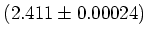GeV) where the 240 keV uncertainty correspond to the 480 keV FWHM of the Froissart-Stora fit. The distinction between resonance and mirror could not be done, because the beam was dumped due to a shutter interlock shortly before the end of shift.

A week later this run was repeated but we planned to reduce dwell and kicker strength in order to receive a more narrow dip, thus increasing certainty of the energy calibration. After observing build-up and correcting the orbit a sweep from 536 kHz to 579 kHz was carried out. When the orbit had been checked to have no deviation form the flat orbit we noted the RF main frequency to be at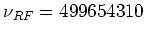Hz. The resonance dip was found at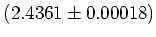GeV (mirror at: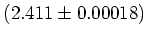GeV) as shown in figure 20.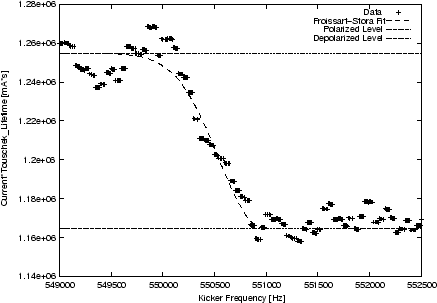Figure 20: Froissart-Stora fit of the depolarizing resonance.

In a second step the RF main frequency was changed by +1000 Hz to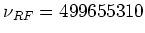Hz. Now the orbit had larger mean values in the arcs in agreement with theory. According to the orbit correction program of the SLS control system (see equation 52)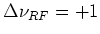kHz leads to an energy deviation of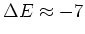MeV. But actually the reproduced dip was now found 7 MeV above the original 2.4361 GeV. This proves that 2.4361 GeV is the actual resonance and not the mirror; the mirror of this resonance would be seen if sweeping around the mirror frequency of 491 kHz. The reason for this conclusion lies in the fact that 2.4361 GeV already lies above the half-integer spin tune (520.8 kHz or 2.4236 GeV), so in the sweeps around this frequency we have been looking at mirror frequencies. If the energy belonging to the shifted RF voltage lies higher even though it should be lower than the energy belonging to the unshifted RF voltage, we are looking at the mirror of a mirror, i.e. we are looking at the actual resonance! Therefore we will from now on link all resonances in sweeps from frequencies above the half-integer to energies above 2.4236 GeV. Figure 21 shows the result of these two runs.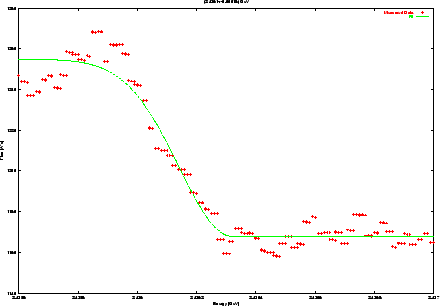Figure 21: The depolarizing resonance dip corresponding to a beam energy ofGeV.

In the last run we planned to reproduce the results of the previous runs but with higher accuracy. We therefore lower the kicker power from 250 W to 50 W and set the dwell to only 2 Hz/s. We were able to reproduce the resonance but at a much higher energy of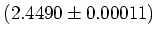GeV as shown in figure 22.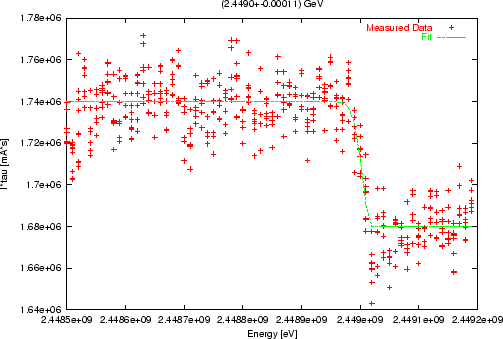Figure 22: The depolarizing resonance dip corresponding to a beam energy ofGeV.

The reason for this sudden energy drift'' however has nothing to do with the actual beam energy as we were able to show later. During the measurement the only peculiarity observed was an exceptionally low RF main frequency of 499653209 Hz; this value was about 1 kHz lower than in previous runs. After carefully studying the events of this night shift it was recognized that the orbit was flat and centered, but that there was a mean corrector setting leading to an orbit which was too far inside of the ring with respect to the design center. Because there was a systematic shift to smaller radii, the RF main frequency was lowered by the orbit correction to smaller values, thus increasing the actual beam energy. It is important to notice that not only does the beam have to be on the reference orbit for the energy calibration to render decent values, but also the correctors pattern is not allowed to have a mean value in the dispersive regions (in the arcs). If the mean corrector value is zero the orbit is centered with respect to the design orbit and energy calibration measurements lead to valid results. In other cases the orbit correction will start shifting the RF main frequency and thus will shift the beam energy.

Notice however that the uncertainty of the last run is already as low as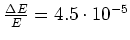meaning that the precision of the energy calibration measurement can be increased with the lower kicker power and dwell settings as described above. Further runs are expected to reach a precision of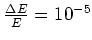!

Finally, we have measured the energy of the SLS storage ring to beGeV and its stability over a couple of weeks is constant within. This is in good agreement with independent energy calibration measurements performed by experimenters in one of the SLS beam lines ; by looking at characteristic line spectra behind undulator U24 (gap at 8 mm) the energy was calibrated at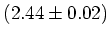GeV which is in excellent agreement to the presented measurements.Next: Outlook Up: Measurement Results Previous: Beam Polarization Level   Contents
Simon Leemann
2002-03-15